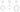← Go back

# Random Numbers in Java

2 years ago by Michael Röder

A variety of algorithms ranging from complex probabilistic models to simple preprocessing steps, (such as sampling a subset of a larger dataset), are based on random numbers. However, our modern computers are deterministic machines. A computer cannot generate a random number unless it contains special hardware that observes a natural random process. To this end, most programs make use of pseudo-random numbers. In this blog post, we want to discuss the algorithm used to generate these numbers in Java and highlight the practical usefulness of seed values. In a second blog post, we will focus on the use of seed values within scalable applications.

### Random number generator

The Random class is the basic random number generator (RNG) class available in Java. For special cases, there are more complex and more secure generators. However, for most cases, this generator is sufficient. It belongs to the class of linear congruential generators, which use the following formula to generate a new random number ni+1 based on the previous number ni:

ni+1= (a × ni + c) mod m

The initial n0 is called the seed. Depending on the different parameters a, c and m, and the chosen seed, a linear congruential generator will serve numbers in a deterministic order and sooner or later end up with the number it started with. Hence, the random numbers can be imagined to be part of a cycle. The RNG starts at one of these numbers and always moves forward on this cycle. The following figure shows three example configurations for a random number generator to randomly choose a number from 0 to 8.The examples show the influence of the parameters and the seed value. The second example uses an unfortunate combination of parameters and seed value, leading to a short cycle. Such a seed is called “weak” and should be avoided.

In an open implementation of the Random class, the values a = 25214903917 and c = 11 are used. The parameter m is given by the user when requesting a new number. However, it is important to note that although the Random class itself works with 64-bit numbers, the random numbers are shortened to 48 bits. In addition, it is known that the high bits of the random numbers are “more random” than the lower bits. The random class does not rely on a modulo operation to apply m as it would prefer lower bits. Instead, the Random class removes lower bits in favor of higher bits to meet the range defined by m.

### Seeds

As pointed out before, the seed has an influence on the quality of random numbers. However, using seed values has additional advantages. First, it is important to note that a typical RNG implementation always relies on a seed value. The RNG must enter the cycle of numbers at a certain point, and this point is defined by the seed value. If an RNG is created without a seed value, it is up to the developer of the RNG implementation to choose a seed value (e.g., the current time on the computer’s clock).

Second, the usage of seed values ensures that two RNG instances initialized with the same seed produce the same random numbers in the same order. This can become beneficial as soon as a program that relies on an RNG produces unexpected results. Without using a seed value, the chance of being able to reproduce the unexpected behavior is very low (1:248 for choosing the same seed again).

### Short Summary

Summarizing the insights from above leads to the following recommendations:

1. Use seeds:
If you use an RNG, make sure to use a seed to initialize it!
2. Allow others to use seeds:
If you write a piece of code that should be used by others and your implementation makes use of an RNG, make sure your code offers the possibility to define the RNG seed.
3. Log seeds:
Recommendations 1 and 2 can lead to a situation in which you (or the user of your code) do not want to define a seed. In this situation, it makes sense to choose a seed (e.g., the current time on the computer’s clock) and log it.
``````// Get seed value
long seed = System.currentTimeMillis();
// Create RNG using the seed value
Random random = new Random(seed);
// Log the seed
logger.debug("Created RNG with seed={}", seed);
``````

This eases the reproduction of results and, hence, the debugging of software.

### What is next?

In our second part of this blog post, we will look at using seeds in more complex setups.